# First-order first-degree differential equation

## Definition

### In implicit form

A first-order first-degree differential equation is a differential equation that is both a first-order differential equation and a first-degree differential equation. Explicitly, it has the form: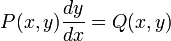$P(x,y) \frac{dy}{dx} = Q(x,y)$

where$P,Q$ are known functions. Here,$x$ is the independent variable and$y$ is the dependent variable.

### In explicit form

Any first-order first-degree differential equation can be converted to an (almost) equivalent first-order explicit differential equation, i.e., a differential equation of the form:$\frac{dy}{dx} = G(x,y)$

### Conversion between the forms

A first-order first-degree differential equation can be converted to explicit form as follows. Start with:$P(x,y) \frac{dy}{dx} = Q(x,y)$

Now, divide both sides by$P(x,y)$ and set$G(x,y) := Q(x,y)/P(x,y)$, giving the explicit form.

Note that the process may involve some slight change in the set of solutions. In particular, any solution that identically satisfies both$P(x,y) = 0$ and$Q(x,y) = 0$ may be lost when we divide. In most cases, there are no such solutions, and there are usually at most finitely many such solutions.

## Existence and uniqueness of solutions

• Peano existence theorem guarantees the existence of a local solution to any initial value problem for an explicit first-order first-degree differential equation$\frac{dy}{dx} = G(x,y)$ with initial value point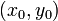$(x_0,y_0)$ provided that$G$ is continuous.
• Picard-Lindelof theorem establishes existence and uniqueness under somewhat stronger continuity and differentiability assumptions.

### Solution strategies in particular cases

Below are some formats of equations for which general strategies are known. Note that the letter$f$ is no longer used for the solution function but may be used for other functions:

Equation type Quick summary of solution strategy
first-order linear differential equation which in simplified form looks like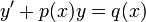$y' + p(x)y = q(x)$ Use the integrating factor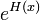$e^{H(x)}$ where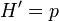$H'=p$. The general solution is$y = Ce^{-H(x)} + e^{-H(x)}\int p(x)e^{H(x)} \, dx$
separable differential equation which is of the form$y' = f(x)g(y)$ (any first-order first-degree autonomous differential equation is separable, though there are separable differential equations that aren't autonomous) Separate and solve as$\int \frac{dy}{g(y)} = \int f(x) \, dx$. Also find solutions corresponding to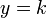$y = k$ where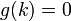$g(k) = 0$.
first-order exact differential equation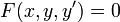$F(x,y,y') = 0$ Try to find a relation$R(x,y)$ such that$F(x,y,y') = \frac{d}{dx}[R(x,y)]$ using implicit differentiation. Finding the$R$, even if it does exist, can be tricky.
Bernoulli differential equation$y' + p(x)y = q(x)y^n$ (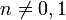$n \ne 0,1$) Divide both sides by$y^n$ (set aside possible stationary solution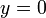$y = 0$), then substitute$w = 1/y^{n-1}$ to get a first-order linear differential equation with dependent variable$w$ and independent variable$x$.